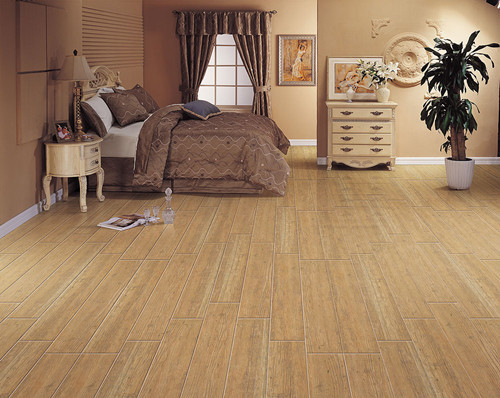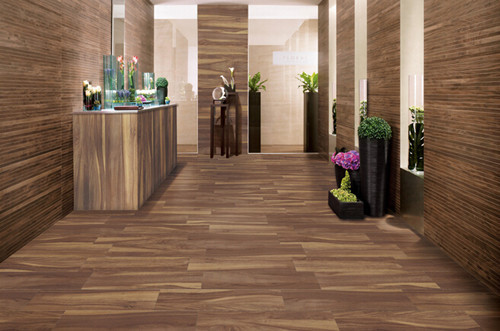|

# 小编揭晓2016木纹砖十大品牌排名

木纹砖是近年来比较受消费者青睐的装饰建材之一，然而随着人们对生活品质要求的逐渐提高，对木纹砖的质量要求也越来越高，现在市面上的木纹砖品牌虽然价格高，但是销量还是能超过知名度不高的产品。因此，接下来小编为大家揭晓2016木纹砖十大品牌排名。2016木纹砖十大品牌排名一、康拓陶瓷

“康拓木纹砖”自面世以来更是受到行业内外一直好评，经过康拓人几年的不懈努力，现已成为行业内公认的“木纹砖首选品牌”。曾先后被评为“中国陶瓷工业协会年度优秀创意企业”、“中国陶瓷工业协会年度最佳新锐产品”、“中国建筑卫生陶瓷十大创新策划品牌”、“中国陶瓷行业新锐榜优秀企业管理文化”、“世博会石油馆制定瓷砖”等殊荣。

2016木纹砖十大品牌排名二、路易摩登陶瓷

路易摩登是争创中国陶瓷产业链领跑者的广东新明珠陶瓷集团旗下主力品牌。自2005年以来，路易摩登陶瓷以强大的研发实力、庞大的生产规模、先进的生产设备、专业的营销团队、鲜明的精英生活主张等优势，迅速成长为中国具有影响力的瓷砖品牌，被誉为建陶行业“瓷质仿古砖”的典范，并与恒大、万科、保利等大型地产公司成为战略合作伙伴。

2016木纹砖十大品牌排名三、新中源陶瓷

被业界誉为建陶航母的新中源陶瓷在深入调研市场，全方位了解消费者的需求之后，凭借其强大的研发能力，再次主动出击推出新品—“臻木”系列瓷质木地板。她是自然界的天然肌理与陶瓷技术的完美碰撞与融合，是新中源继V7微晶石、臻冠·绝色控之后新产品中的又一颗璀璨新星，将自然搬回家，尊享纯粹木之味。

2016木纹砖十大品牌排名四、金意陶陶瓷

森活木，是金意陶独创的一个瓷砖品种。它珍选以往普通人只听其名，未闻其质的王室御用木材金丝楠木，比黄金还贵重的海南花梨木，有木中极品的印度小叶紫檀，饱经岁月蚀刻的老船木等世界各地的名贵木材为模板，通过数字化技术完整采集名木的花色、质地，萃取制作成标本，再通过高清喷墨打印、数字雕模、多层叠釉等原生映像技术，把标本映刻在瓷砖上。

2016木纹砖十大品牌排名五、ICC陶瓷

ICC整合了Interceramic30多年国际市场产品开发及管理经验，以及Interceramic旗下25家研发中心、9大生产基地带来的世界一流的设备、工艺、研发体系，同时汲取了金意陶仿古砖领域的优势，融汇了欧美现代艺术精华，并创造性地融入了东方意境的描绘，以崭新的空间设计理念，打造出“集萃中西智汇全球”的全新高端陶瓷品牌。2016木纹砖十大品牌排名六、罗马利奥瓷砖

罗马利奥瓷砖，1999年品牌缔造于中国陶瓷之都佛山，是广东冠星陶瓷企业集团旗下核心品牌。经过多年创新发展，罗马利奥品牌现已发展成为以仿古砖产品为核心的全品类综合性高端建筑陶瓷品牌。

2016木纹砖十大品牌排名七、懋隆陶瓷

成立于2008年的佛山市懋隆陶瓷有限公司，位于有“南国陶都”之称的佛山，是一家集科研、开发、生产、销售、多元化经营于一体的大型现代化陶瓷生产企业。公司旗下的强势品牌——懋隆瓷砖，荣获“广东省木纹砖前三甲品牌”、“全国消费者放心满意品牌”等诸多荣誉称号。

2016木纹砖十大品牌排名八、新中源陶瓷

被业界誉为建陶航母的新中源陶瓷在深入调研市场，全方位了解消费者的需求之后，凭借其强大的研发能力，再次主动出击推出新品—“臻木”系列瓷质木地板。她是自然界的天然肌理与陶瓷技术的完美碰撞与融合，是新中源继V7微晶石、臻冠·绝色控之后新产品中的又一颗璀璨新星，将自然搬回家，尊享纯粹木之味。

2016木纹砖十大品牌排名九、金意陶陶瓷

森活木，是金意陶独创的一个瓷砖品种。它珍选以往普通人只听其名，未闻其质的王室御用木材金丝楠木，比黄金还贵重的海南花梨木，有木中极品的印度小叶紫檀，饱经岁月蚀刻的老船木等世界各地的名贵木材为模板，通过数字化技术完整采集名木的花色、质地，萃取制作成标本，再通过高清喷墨打印、数字雕模、多层叠釉等原生映像技术，把标本映刻在瓷砖上。

2016木纹砖十大品牌排名十、陶木然瓷砖

陶木然是一家致力于为全球消费者提供更贴近自然的建陶产品的现代化大型企业。从创立之初便专注于木纹砖等原生态风格产品的设计、研发及生产，经过有效的运作与时间的沉淀逐步奠定了陶木然在行业内的知名品牌地位。

编辑总结：关于2016木纹砖十大品牌排名就介绍到这里了，希望对大家有所帮助。想了解更多相识，可以关注网资讯。

`声明：本文由入驻焦点开放平台的作者撰写，除焦点官方账号外，观点仅代表作者本人，不代表焦点立场错误信息举报电话： 400-099-0099，邮箱：jubao@vip.sohu.com，或点此进行意见反馈，或点此进行举报投诉。`A B C D E F G H J K L M N P Q R S T W X Y Z
A - B - C - D - E
• A
• 鞍山
• 安庆
• 安阳
• 安顺
• 安康
• 澳门
• B
• 北京
• 保定
• 包头
• 巴彦淖尔
• 本溪
• 蚌埠
• 亳州
• 滨州
• 北海
• 百色
• 巴中
• 毕节
• 保山
• 宝鸡
• 白银
• 巴州
• C
• 承德
• 沧州
• 长治
• 赤峰
• 朝阳
• 长春
• 常州
• 滁州
• 池州
• 长沙
• 常德
• 郴州
• 潮州
• 崇左
• 重庆
• 成都
• 楚雄
• 昌都
• 慈溪
• 常熟
• D
• 大同
• 大连
• 丹东
• 大庆
• 东营
• 德州
• 东莞
• 德阳
• 达州
• 大理
• 德宏
• 定西
• 儋州
• 东平
• E
• 鄂尔多斯
• 鄂州
• 恩施
F - G - H - I - J
• F
• 抚顺
• 阜新
• 阜阳
• 福州
• 抚州
• 佛山
• 防城港
• G
• 赣州
• 广州
• 桂林
• 贵港
• 广元
• 广安
• 贵阳
• 固原
• H
• 邯郸
• 衡水
• 呼和浩特
• 呼伦贝尔
• 葫芦岛
• 哈尔滨
• 黑河
• 淮安
• 杭州
• 湖州
• 合肥
• 淮南
• 淮北
• 黄山
• 菏泽
• 鹤壁
• 黄石
• 黄冈
• 衡阳
• 怀化
• 惠州
• 河源
• 贺州
• 河池
• 海口
• 红河
• 汉中
• 海东
• I
• J
• 晋中
• 锦州
• 吉林
• 鸡西
• 佳木斯
• 嘉兴
• 金华
• 景德镇
• 九江
• 吉安
• 济南
• 济宁
• 焦作
• 荆门
• 荆州
• 江门
• 揭阳
• 金昌
• 酒泉
• 嘉峪关
K - L - M - N - P
• K
• 开封
• 昆明
• 昆山
• L
• 廊坊
• 临汾
• 辽阳
• 连云港
• 丽水
• 六安
• 龙岩
• 莱芜
• 临沂
• 聊城
• 洛阳
• 漯河
• 娄底
• 柳州
• 来宾
• 泸州
• 乐山
• 六盘水
• 丽江
• 临沧
• 拉萨
• 林芝
• 兰州
• 陇南
• M
• 牡丹江
• 马鞍山
• 茂名
• 梅州
• 绵阳
• 眉山
• N
• 南京
• 南通
• 宁波
• 南平
• 宁德
• 南昌
• 南阳
• 南宁
• 内江
• 南充
• P
• 盘锦
• 莆田
• 平顶山
• 濮阳
• 攀枝花
• 普洱
• 平凉
Q - R - S - T - W
• Q
• 秦皇岛
• 齐齐哈尔
• 衢州
• 泉州
• 青岛
• 清远
• 钦州
• 黔南
• 曲靖
• 庆阳
• R
• 日照
• 日喀则
• S
• 石家庄
• 沈阳
• 双鸭山
• 绥化
• 上海
• 苏州
• 宿迁
• 绍兴
• 宿州
• 三明
• 上饶
• 三门峡
• 商丘
• 十堰
• 随州
• 邵阳
• 韶关
• 深圳
• 汕头
• 汕尾
• 三亚
• 三沙
• 遂宁
• 山南
• 商洛
• 石嘴山
• T
• 天津
• 唐山
• 太原
• 通辽
• 铁岭
• 泰州
• 台州
• 铜陵
• 泰安
• 铜仁
• 铜川
• 天水
• 天门
• W
• 乌海
• 乌兰察布
• 无锡
• 温州
• 芜湖
• 潍坊
• 威海
• 武汉
• 梧州
• 渭南
• 武威
• 吴忠
• 乌鲁木齐
X - Y - Z
• X
• 邢台
• 徐州
• 宣城
• 厦门
• 新乡
• 许昌
• 信阳
• 襄阳
• 孝感
• 咸宁
• 湘潭
• 湘西
• 西双版纳
• 西安
• 咸阳
• 西宁
• 仙桃
• 西昌
• Y
• 运城
• 营口
• 盐城
• 扬州
• 鹰潭
• 宜春
• 烟台
• 宜昌
• 岳阳
• 益阳
• 永州
• 阳江
• 云浮
• 玉林
• 宜宾
• 雅安
• 玉溪
• 延安
• 榆林
• 银川
• Z
• 张家口
• 镇江
• 舟山
• 漳州
• 淄博
• 枣庄
• 郑州
• 周口
• 驻马店
• 株洲
• 张家界
• 珠海
• 湛江
• 肇庆
• 中山
• 自贡
• 资阳
• 遵义
• 昭通
• 张掖
• 中卫

1室1厅1厨1卫1阳台

1
2
3
4
5

0
1
2

1

1

0
1
2
3报名成功，资料已提交审核A B C D E F G H J K L M N P Q R S T W X Y Z
A - B - C - D - E
• A
• 鞍山
• 安庆
• 安阳
• 安顺
• 安康
• 澳门
• B
• 北京
• 保定
• 包头
• 巴彦淖尔
• 本溪
• 蚌埠
• 亳州
• 滨州
• 北海
• 百色
• 巴中
• 毕节
• 保山
• 宝鸡
• 白银
• 巴州
• C
• 承德
• 沧州
• 长治
• 赤峰
• 朝阳
• 长春
• 常州
• 滁州
• 池州
• 长沙
• 常德
• 郴州
• 潮州
• 崇左
• 重庆
• 成都
• 楚雄
• 昌都
• 慈溪
• 常熟
• D
• 大同
• 大连
• 丹东
• 大庆
• 东营
• 德州
• 东莞
• 德阳
• 达州
• 大理
• 德宏
• 定西
• 儋州
• 东平
• E
• 鄂尔多斯
• 鄂州
• 恩施
F - G - H - I - J
• F
• 抚顺
• 阜新
• 阜阳
• 福州
• 抚州
• 佛山
• 防城港
• G
• 赣州
• 广州
• 桂林
• 贵港
• 广元
• 广安
• 贵阳
• 固原
• H
• 邯郸
• 衡水
• 呼和浩特
• 呼伦贝尔
• 葫芦岛
• 哈尔滨
• 黑河
• 淮安
• 杭州
• 湖州
• 合肥
• 淮南
• 淮北
• 黄山
• 菏泽
• 鹤壁
• 黄石
• 黄冈
• 衡阳
• 怀化
• 惠州
• 河源
• 贺州
• 河池
• 海口
• 红河
• 汉中
• 海东
• I
• J
• 晋中
• 锦州
• 吉林
• 鸡西
• 佳木斯
• 嘉兴
• 金华
• 景德镇
• 九江
• 吉安
• 济南
• 济宁
• 焦作
• 荆门
• 荆州
• 江门
• 揭阳
• 金昌
• 酒泉
• 嘉峪关
K - L - M - N - P
• K
• 开封
• 昆明
• 昆山
• L
• 廊坊
• 临汾
• 辽阳
• 连云港
• 丽水
• 六安
• 龙岩
• 莱芜
• 临沂
• 聊城
• 洛阳
• 漯河
• 娄底
• 柳州
• 来宾
• 泸州
• 乐山
• 六盘水
• 丽江
• 临沧
• 拉萨
• 林芝
• 兰州
• 陇南
• M
• 牡丹江
• 马鞍山
• 茂名
• 梅州
• 绵阳
• 眉山
• N
• 南京
• 南通
• 宁波
• 南平
• 宁德
• 南昌
• 南阳
• 南宁
• 内江
• 南充
• P
• 盘锦
• 莆田
• 平顶山
• 濮阳
• 攀枝花
• 普洱
• 平凉
Q - R - S - T - W
• Q
• 秦皇岛
• 齐齐哈尔
• 衢州
• 泉州
• 青岛
• 清远
• 钦州
• 黔南
• 曲靖
• 庆阳
• R
• 日照
• 日喀则
• S
• 石家庄
• 沈阳
• 双鸭山
• 绥化
• 上海
• 苏州
• 宿迁
• 绍兴
• 宿州
• 三明
• 上饶
• 三门峡
• 商丘
• 十堰
• 随州
• 邵阳
• 韶关
• 深圳
• 汕头
• 汕尾
• 三亚
• 三沙
• 遂宁
• 山南
• 商洛
• 石嘴山
• T
• 天津
• 唐山
• 太原
• 通辽
• 铁岭
• 泰州
• 台州
• 铜陵
• 泰安
• 铜仁
• 铜川
• 天水
• 天门
• W
• 乌海
• 乌兰察布
• 无锡
• 温州
• 芜湖
• 潍坊
• 威海
• 武汉
• 梧州
• 渭南
• 武威
• 吴忠
• 乌鲁木齐
X - Y - Z
• X
• 邢台
• 徐州
• 宣城
• 厦门
• 新乡
• 许昌
• 信阳
• 襄阳
• 孝感
• 咸宁
• 湘潭
• 湘西
• 西双版纳
• 西安
• 咸阳
• 西宁
• 仙桃
• 西昌
• Y
• 运城
• 营口
• 盐城
• 扬州
• 鹰潭
• 宜春
• 烟台
• 宜昌
• 岳阳
• 益阳
• 永州
• 阳江
• 云浮
• 玉林
• 宜宾
• 雅安
• 玉溪
• 延安
• 榆林
• 银川
• Z
• 张家口
• 镇江
• 舟山
• 漳州
• 淄博
• 枣庄
• 郑州
• 周口
• 驻马店
• 株洲
• 张家界
• 珠海
• 湛江
• 肇庆
• 中山
• 自贡
• 资阳
• 遵义
• 昭通
• 张掖
• 中卫• 手机• 分享
• 设计
免费设计
• 计算器
装修计算器
• 入驻
合作入驻
• 联系
联系我们
• 置顶
返回顶部